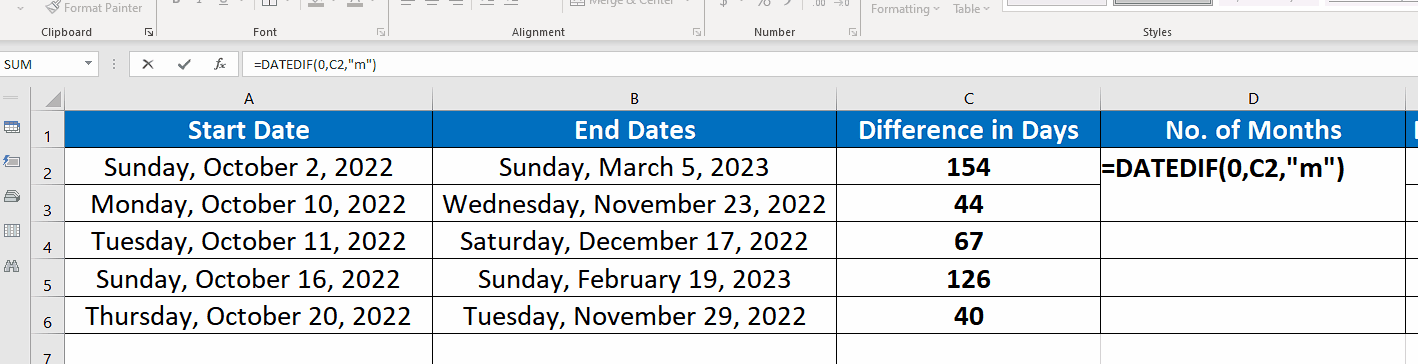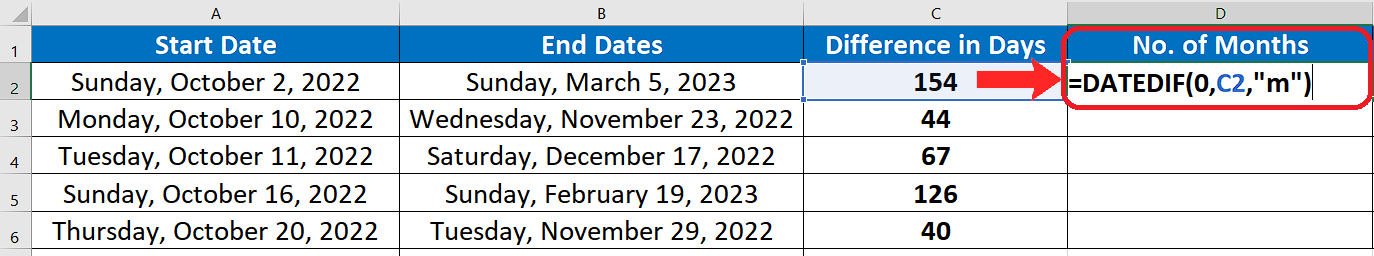# How to convert number of days between two dates to months in Excel

## Structure of DATEDIF

The standard syntax of countif function in excel is shown below;

=DATEDIF(start_date, end_date, unit)

• start_date

Could be any date earlier than the second parameter end date. Otherwise errors will occur.

• end_date

Could be any date earlier than the second parameter end date. Otherwise errors will occur.

• unit

“y” It will calculate the number of years between the specified period

“m” It will calculate the number of months between the specified period

“d” It will calculate the number of days between the specified period

Implementing the Formula on an Actual Data Set

We can see in the example data set that there are some start dates that are earlier than the end dates and we have already calculated the number of days between these dates.

Let’s convert the number of days between start date and end date to months by following these steps;Counting the number of months between two dates while working with dates data is quite a common thing. Excel provides a variety of formulas for working with the dates. Sometimes we get a requirement to convert days between two dates to months.

Excel has the built-in function to calculate the months between the number of days through the “DATEDIF” function. However, this formula is not included in the standard formula list by Excel. Which means that when you type this formula Excel will not show its help just like it does while typing in other standard formulas. So it’s kind of a hidden (secret) formula. The best way to understand how DATEDIF works is to first understand the structure of the function and the parameters it requires.

### Step 1 – Create the appropriate formula:– Choose a suitable cell where you wish to implement the formula. In our example it is C2.

– Use the following formula in that cell and press enter key.

=DATEDIF(0,C2,”m”)

### Step 2 – Implement the formula to convert Day to Months:– Now we will implement the formula to convert the days between two dates to the number of months between two dates. After writing the formula just press the enter key. The result will be displayed in the cell. To extend the formula drag down the formula as shown in the figure.

– So by using Excel’s DATEDIF function we can easily find the difference of months between two dates.